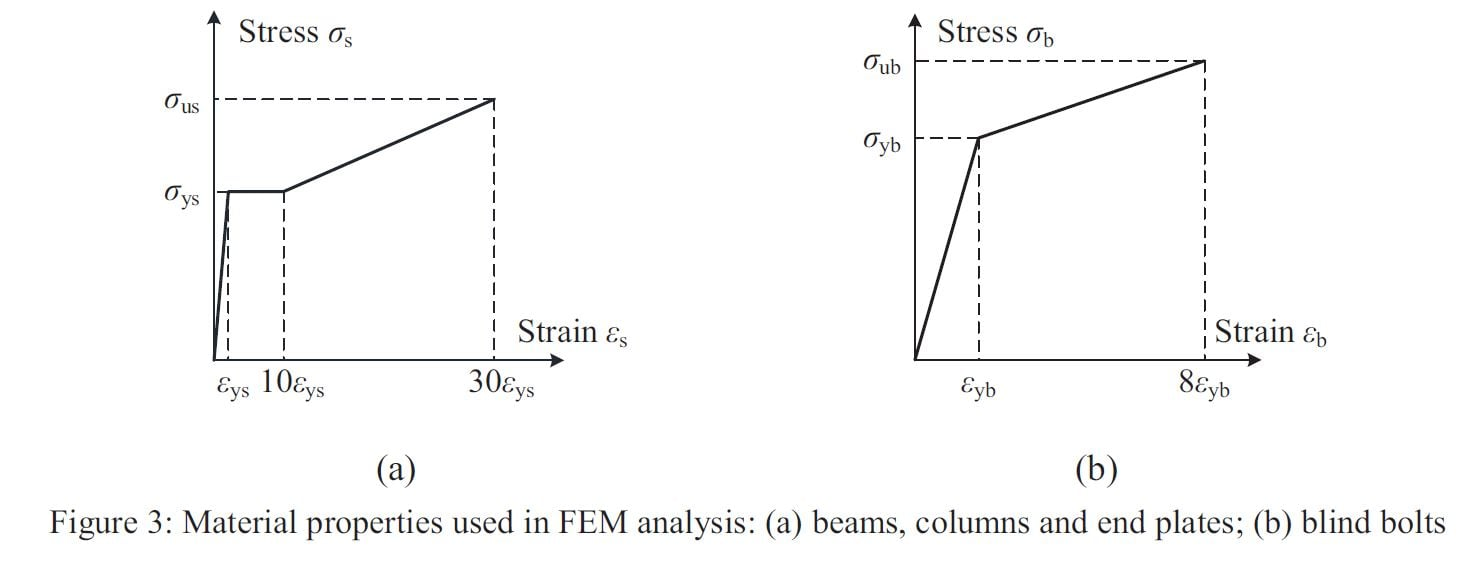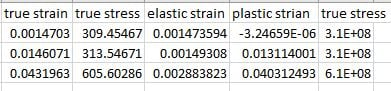## General Mechanical

•kartik
Subscriber

hi, i'm having a bit trouble in converting the engineering stress- strain curve to true stress - strain curve. after converting the values and inputting them in multi-linear isotropic hardening the solution is not converging. (i converted to plastic strain and true stress, this input i had given in the engg data).

could anyone give a in detail procedure for inputting the material data from raw engg stress strain curve.

•peteroznewman
Subscriber
•Sandeep Medikonda
Ansys Employee

Kartik,

What is the error message that you are seeing which is stopping the solution from converging?

Make sure that the max. plastic strain in your simulation is less than what you have input for the strain in the engineering data.

~Sandeep

•kartik
Subscriber

in that you have mentioned , the first line is yield stress. i didnt understand that,

i have followed the same steps from that post earlier but the values after inputting is not converging.

if you dont mind can you please just have a look at it and see if it is correct.

•kartik
Subscriber

solver failed to converge

•Sandeep Medikonda
Ansys Employee

Kartik,

Yield stress is the value on your stress-strain plot beyond which the material starts to deform plastically, i.e., the deformation is permanent. Please post snapshots of your settings/model/error messages?

Here is a document that you might find helpful.

~Sandeep

•Ayman
Subscriber

i  study model with non stress strain curve how could i define it in ansys material (multi linear isotropic hardening)when calculate these values:

True Strain = ln(1+Strain)   where ln is the natural logarithm function.

True Stress = Stress(1+Strain)

Elastic Strain = True Stress/E

Plastic Strain = True Strain - Elastic Strain

where:

fy=309 mpa

fu= 580 mpa

E= 210 gpa

i get:but the deformation decreases from 0.006 to 0.001 and still solve linear i don't know what is the problem